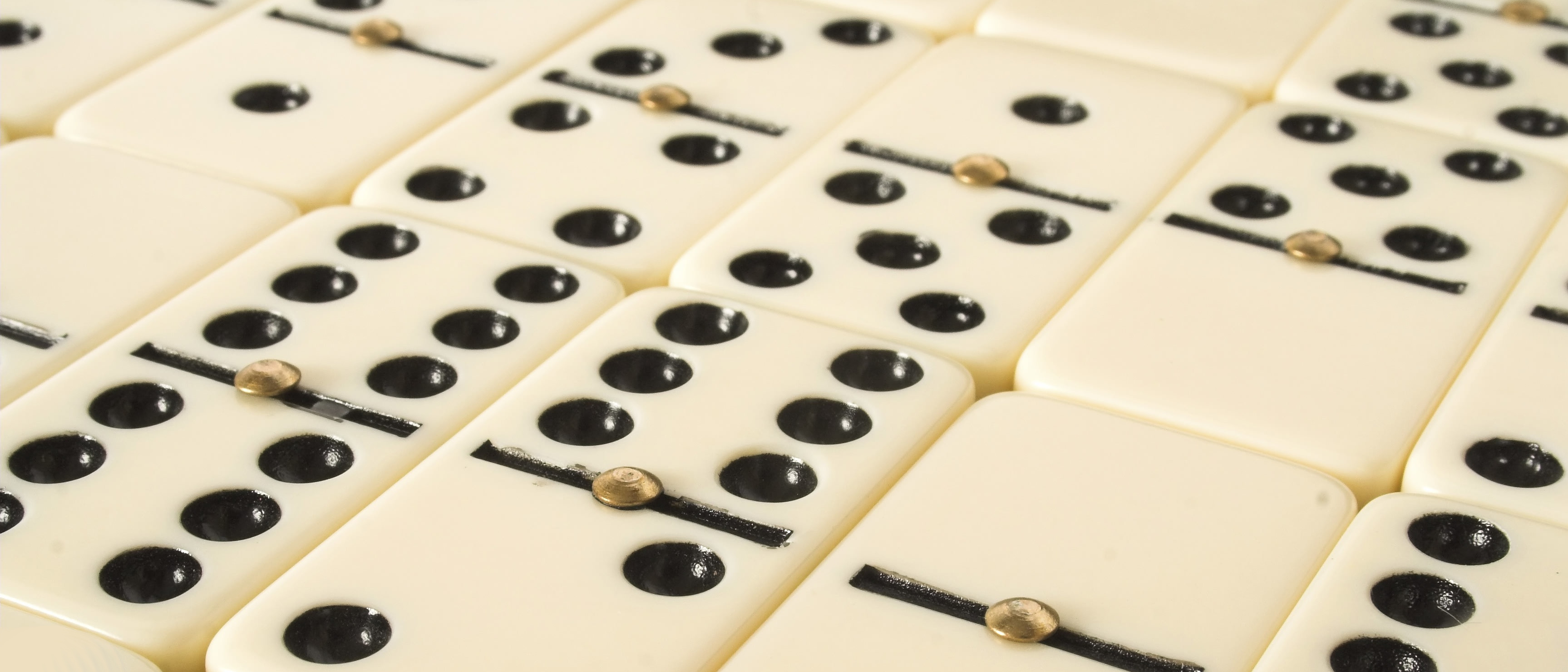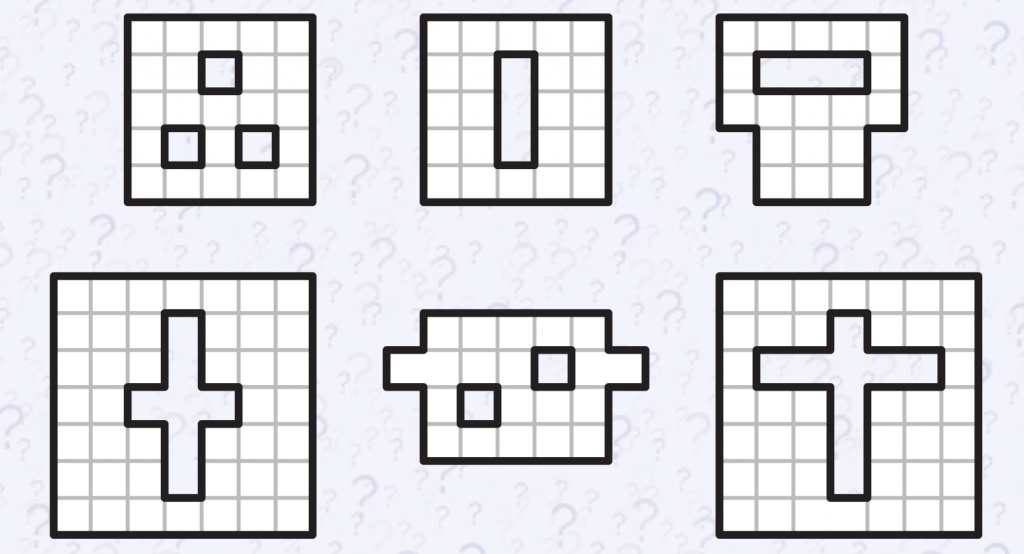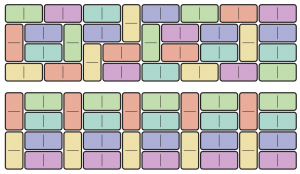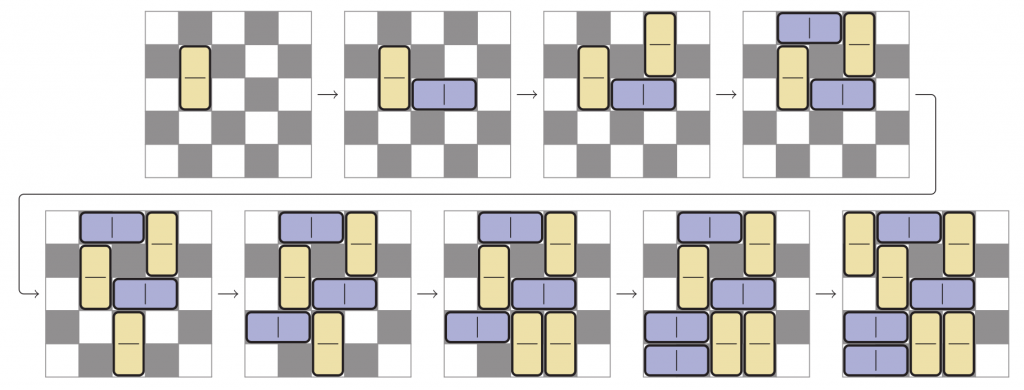# Domino tiling & domineering

John Dore and Chris Woodcock join the dotsProbably everyone reading this article has, perhaps some time ago, played dominoes with the standard set of 28 pieces or even with the larger set of 55 pieces. But do not fret if you haven’t: we will not be examining these sets, but merely taking the concept of a domino as a couple of squares joined along an edge.

Asking how we may arrange a set of pieces to cover a plane rectangular board leads to an interesting mathematical problem. If we add some orientation restrictions, we can make an interesting two-player game.

## Domino tilingA chessboard with two opposite corners removed

There is a standard problem in many recreational maths books in which two opposite corners of a chessboard are removed and the reader is asked to place dominoes to cover all the remaining squares of the board.

It is, of course, impossible because the two squares removed have the same colour and each of the dominoes must cover one black square and one white square. However, there is no need to introduce this somewhat contrived condition in order to obtain some relatively simple, but
interesting mathematical problems.

## Problem 1

How many different ways can eight dominoes be used to cover a $4\times4$ square?For problem 2, these two tilings are considered the same, as one is a rotation of the other

Alternatively, tilings may be considered the same if one is a rotation of the other, but still regarded as different if they are mirror images. This change in counting the distinct tilings obviously leads to a smaller number due to symmetry, but there is no simple means of calculating the different possibilities as some patterns have two-fold rotational symmetry and others involving squares may have four-fold rotational symmetry. However, it does provide a nice problem to be examined through a systematic `trial-and-error’ approach:

## Problem 2

How many different ways can eight dominoes be used to cover a $4\times4$ square, if rotations of the same pattern are excluded?

We now return to including all the rotations, as in problem 1, take a more general $m\times n$ rectangle and ask a similar question. This problem has troubled mathematicians and the full answer is quite complicated. It was solved in 1961 by Kasteleyn in the context of covering a surface with molecular dimers, and independently solved by Temperley and Fisher in the same year. The resulting equation for the total number of domino tilings of an $m\times n$ rectangular board can be shown to be

$$A_{m,n}:=\left(\prod_{k=1}^n\left(\prod_{j=1}^m\left(4\cos\left(\frac{\mathrm{\pi} j}{m+1}\right)^2+4\cos\left(\frac{\mathrm{\pi} k}{n+1}\right)^2\right)\right)\right)^{1/4}.$$The 5 ways of tiling a $2\times4$ rectangle

Perhaps surprisingly, this product must therefore always be a non-negative integer! Although we may use this expression to calculate the numbers for various rectangles, it is perhaps more interesting to examine a few specific cases.

We begin by looking at the problem for a $2\times n$ rectangle. As you will see, this has a surprisingly familiar answer. The first few cases with small $n$ can be easily worked out and the answers are:

 $n$ 1 2 3 4 5 6 $A(n)$ 1 2 3 5 8 13

Let us now assume that we know the answer, $A(n)$, for some small values of $n$. We see that we can generate the ways of tiling a $2\times(n+1)$ rectangle in two different ways: either by adding a vertical domino to one of our ways of tiling a $2\times n$ rectangle; or by adding two horizontal dominoes to one of our ways of tiling a $2\times(n-1)$ rectangle.Consequently, we may write $A(n+1)=A(n)+A(n-1)$ and, since we already know $A(1)=1$ and $A(2)=2$, we have a recurrence relation that generates the sequence that we saw above, and that you will immediately recognise as the Fibonacci sequence. We suspect that you didn’t expect it to make an appearance in this context.

Of course, it’s not necessary to restrict ourselves to rectangles, so here are a few more shapes to consider:

## Problem 3

Which of the following figures are capable of being completely tiled by dominoes?Returning swiftly to rectangles, we note that the $2\times n$ rectangle has an interesting solution. This immediately raises a further question: is it possible to similarly analyse the $3\times n$ rectangle? This question is more difficult to address as there are more configurations for the dominoes in the first few columns. One approach has been made by Brundan, but to our knowledge no detailed analysis of the complete solution has been previously published. The way of approaching the problem, along similar lines, is to think in terms of the arrangement of dominoes on the left-hand side of the block and to investigate how these define the number of configurations as the further dominoes are placed on the right-hand side.

If $m = 3$, then clearly there are no tilings unless $n = 2k$ is even. Let $S(\hspace{-1pt}k\hspace{1pt})$ denote the number of tilings of a $3\times2k$ rectangular board. Then $S(0) = 1$ (as there’s only one way to tile a $0\times0$ board: do nothing!) and it is also easily seen that $S(1) = 3$.

In fact, by considering the possible configurations of the dominoes in the leftmost $3\times2$ and $3\times 4$ rectangles, it can be shown that the recurrence relation $S(k) = 4S(k-1) – S(k-2)$ holds for all $k > 1$. Thus $S(2) = 11$, $S(3) = 41$, $S(4) = 153$, and so on. This recurrence relation allows one to determine the number of tilings for any value of $k$. It follows that there are 571 ways to tile a $3\times10$ rectangle. Perhaps you’d like to try drawing all of these to check?

If we only count up inequivalent tilings of an $m\times n$ rectangular board (as we did in problem 2, where we regarded two such tilings as being equivalent if one is a rotation of the other), then the calculation becomes even more intricate! For example, if $m = 2$, then the
number of inequivalent tilings is 1 if $n=2$,
\begin{align*}
&\frac{F\hspace{1pt}(n+1)+F\hspace{1pt}(\tfrac{n+4}2)}2&&\text{if }n>2\text{ and }n\text{ is even, and}\\
&\frac{F\hspace{1pt}(n+1)+F\hspace{1pt}(\tfrac{n+1}2)}2&&\text{if }n\text{ is odd},
\end{align*}
where $F(n)$ is the $n$th Fibonacci number. This result, involving an averaging process, is a special case of Burnside’s lemma from group theory (in this case the group involved is the rotational symmetry group of a rectangle). Thus for a $2\times6$ rectangle, there are $F(7) = 13$ tilings but only $\tfrac12(F(7) + F(5)) = 9$ inequivalent tilings.Two of the 3,335,651 ways of tiling a $4\times15$ rectangle

It is, of course, possible to extend this method to the general case with $m = 4$. It is obviously more difficult to derive the recurrence relation for this condition but it can be done. Various surprising factors occur and the procedure, which is a littleChalkdust website.

Our method gives the answer for a $4\times10$ rectangle as 18,061 and for a $4\times15$ rectangle as 3,335,651. If you disagree with this result please let us know, but please don’t send all the possible tiling patterns to Chalkdust for checking!

## The game of domineering

We now move to a two-player game that uses dominoes. It was invented in 1974 by Goran Andersson, who originally called it crosscram, although it is now more widely known as the game of domineering.

In this game, players take turns to place a domino on a rectangular board but one is restricted to placing them in a vertical orientation (player V) and the other (player H) is restricted to placing them in a horizontal position; it is conventional for player V to start with square boards and this causes no restriction as the board can obviously be rotated by 90°. The first person who is unable to play has lost the game.An example game of domineering on a $5\times5$ board. Player H cannot make another move so has lost this game.

The rules are therefore very simple but the game has a number of interesting aspects that relate to the tiling problem. A quick (around 5 minute) game can be played on a $5\times5$ board in order to learn the tactics. Play on a $6\times6$ board provides some interesting possibilities that can be easily analysed. So, we now present you with problem 4.

## Problem 4

With best play, who wins (a) on the $5\times5$ board and (b) on the $6\times6$ board?

The answers to the puzzles will be given in a few weeks’ time on the Chalkdust blog, when we will reveal a little more about this game. If you require a more challenging game to play and analyse, try domineering on an $8\times8$ board…Chris and John are both at the University of Kent in Canterbury: Chris is a senior lecturer in the School of Mathematical Sciences, and John is a retired physicist, now an emeritus professor in the School of Physical
Sciences. They both have an interest in various aspects of recreational mathematics and are keen to introduce others to the fun aspects of mathematics through games and puzzles. They particularly enjoy
encouraging people to reach that ‘Aha!’ moment, when the correct approach suddenly becomes apparent. Both were keen chess players in their youth but don’t have time to play competitively, these days.